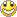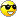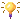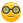Some new stuff
1. Arduino Park Control
2. Arduino USB2serial programming the bare bone
3. Arduino Projects Videos added
4. Arduino Xbee projects
5. Arduino dB Meter
6. Trying to get the IOIO going.
7. IOIO is going....bluetooth8. Attiny 2313 bluetooth bee RC car
9. Compass and temperature added
10. AVR programmer
11. USB2Serial and Ethernet POE
12. Arduino LPG Sensor
13. Program the Arduino Mini 05
14. Lipo Rider Pro in action
15. EZ Robot Builder kit arrived 10 Nov 2012
16. Arduino VA Meter!
17. 05 Nov 2012 Project Sentry Gun.
18. Attiny85 PIR Sensor 10 Dec 2012
19. 0-30VDC3A and 2-28VDC10A bench PSU's20. 19 Dec 2012 Arduino Voltmeter LCD
21. 29 Dec 2012 Arduino Tachometer&Speedometer LCD
22. 03 Jan 2013 Arduino AC Phase Control.
23. 18 Jan 2013 Arduino Distance Meter
24. 22 Jan 2013 Arduino Digital Clock and Date
25. 31 Jan 2013 Arduino Digital Clock and Date 8x2 LCD
26. Feb 2013 LCD and Backpack
27. Feb 2013 7 Segment Serial Clock Sparkfun. Distance Sensor with "newping" library
28. Feb 2013 Lelo Remote
29. 01/03/2013 Arduino Adafruit 7 Seg Digital Clock with RTC and backpack
30. Arduino Frequency Meter 26/03/2013
31. Another RC  Car controlled by Arduino 06/04/2013
32. My quadcopter project update 25 April 2013
33. My Aqua Quad Copter Flying 26 April 201334. Another monster RC truck;strong one! 11July201335. X-Frame Quad Copter 31 July 201336. Arduino GSM Sheild added.28/8/2013
37. 1.8inch TFT screen 13/09/201338. 04/11/2014 added PID Soldering Iron Control
39. Added bluetooth servo control 04/11/2014
40. ESP8266 first try.16/03/2015
41. Arduino Uno Quadcopter 19 May 2015
42. IOT WemosD1 Amp Meter05 Feb 2013

Arduino Altitude and pressure meter LCD 16x2 with Backpack.

Not my code (by: Jim Lindblom  SparkFun Electronics) but I changed the code to accommodate the backpack LCD and experimented with the characters on different places on the LCD like in the photo.

Code:

//SCL = A5

//SCA = A4

//Backpack Adafruit parallel connected with BMP085 sensor

#include "Wire.h"

#include "LiquidCrystal.h"

#define BMP085_ADDRESS 0x77  // I2C address of BMP085

LiquidCrystal lcd(0);

const unsigned char OSS = 0;  // Oversampling Setting

const float p0 = 101325;     // Pressure at sea level (Pa)

float altitude;

// Calibration values

int ac1;

int ac2;

int ac3;

unsigned int ac4;

unsigned int ac5;

unsigned int ac6;

int b1;

int b2;

int mb;

int mc;

int md;

// b5 is calculated in bmp085GetTemperature(...), this variable is also used in bmp085GetPressure(...)

// so ...Temperature(...) must be called before ...Pressure(...).

long b5;

short temperature;

long pressure;

void setup()

{

Serial.begin(9600);

Wire.begin();

bmp085Calibration();

lcd.begin(16,2);

}

void loop()

{

altitude = (float)44330 * (1 - pow(((float) pressure/p0), 0.190295));

lcd.clear();

lcd.setCursor(0,0);

lcd.print("Pres:");

lcd.setCursor(6,0);

lcd.print(pressure,DEC);

lcd.print(" Pa");

lcd.setCursor(0,1);

lcd.print("Alti:");

lcd.setCursor(6,1);

lcd.print(altitude,2);

lcd.setCursor(14,1);

lcd.print("m");

delay(1000);

}

// Stores all of the bmp085's calibration values into global variables

// Calibration values are required to calculate temp and pressure

// This function should be called at the beginning of the program

void bmp085Calibration()

{

}

// Calculate temperature given ut.

// Value returned will be in units of 0.1 deg C

short bmp085GetTemperature(unsigned int ut)

{

long x1, x2;

x1 = (((long)ut - (long)ac6)*(long)ac5) >> 15;

x2 = ((long)mc << 11)/(x1 + md);

b5 = x1 + x2;

return ((b5 + 8)>>4);

}

// Calculate pressure given up

// calibration values must be known

// b5 is also required so bmp085GetTemperature(...) must be called first.

// Value returned will be pressure in units of Pa.

long bmp085GetPressure(unsigned long up)

{

long x1, x2, x3, b3, b6, p;

unsigned long b4, b7;

b6 = b5 - 4000;

// Calculate B3

x1 = (b2 * (b6 * b6)>>12)>>11;

x2 = (ac2 * b6)>>11;

x3 = x1 + x2;

b3 = (((((long)ac1)*4 + x3)<<OSS) + 2)>>2;

// Calculate B4

x1 = (ac3 * b6)>>13;

x2 = (b1 * ((b6 * b6)>>12))>>16;

x3 = ((x1 + x2) + 2)>>2;

b4 = (ac4 * (unsigned long)(x3 + 32768))>>15;

b7 = ((unsigned long)(up - b3) * (50000>>OSS));

if (b7 < 0x80000000)

p = (b7<<1)/b4;

else

p = (b7/b4)<<1;

x1 = (p>>8) * (p>>8);

x1 = (x1 * 3038)>>16;

x2 = (-7357 * p)>>16;

p += (x1 + x2 + 3791)>>4;

return p;

}

// Read 1 byte from the BMP085 at 'address'

{

unsigned char data;

Wire.endTransmission();

while(!Wire.available());

}

// Read 2 bytes from the BMP085

// First byte will be from 'address'

// Second byte will be from 'address'+1

{

unsigned char msb, lsb;

Wire.endTransmission();

while(Wire.available()<2);

return (int) msb<<8 | lsb;

}

// Read the uncompensated temperature value

{

unsigned int ut;

// Write 0x2E into Register 0xF4

// This requests a temperature reading

Wire.write(0xF4);

Wire.write(0x2E);

Wire.endTransmission();

// Wait at least 4.5ms

delay(5);

// Read two bytes from registers 0xF6 and 0xF7

return ut;

}

// Read the uncompensated pressure value

{

unsigned char msb, lsb, xlsb;

unsigned long up = 0;

// Write 0x34+(OSS<<6) into register 0xF4

// Request a pressure reading w/ oversampling setting

Wire.write(0xF4);

Wire.write(0x34 + (OSS<<6));

Wire.endTransmission();

// Wait for conversion, delay time dependent on OSS

delay(2 + (3<<OSS));

// Read register 0xF6 (MSB), 0xF7 (LSB), and 0xF8 (XLSB)

Wire.write(0xF6);

Wire.endTransmission();

// Wait for data to become available

while(Wire.available() < 3);